# Differentiation 3 Basic Rules of Differentiation The Product

• Slides: 31Differentiation 3 • Basic Rules of Differentiation • The Product and Quotient Rules • The Chain Rule • Marginal Functions in Economics • Higher-Order Derivatives • Implicit Differentiation and Related Rates • Differentials Copyright © 2006 Brooks/Cole, a division of Thomson Learning, Inc.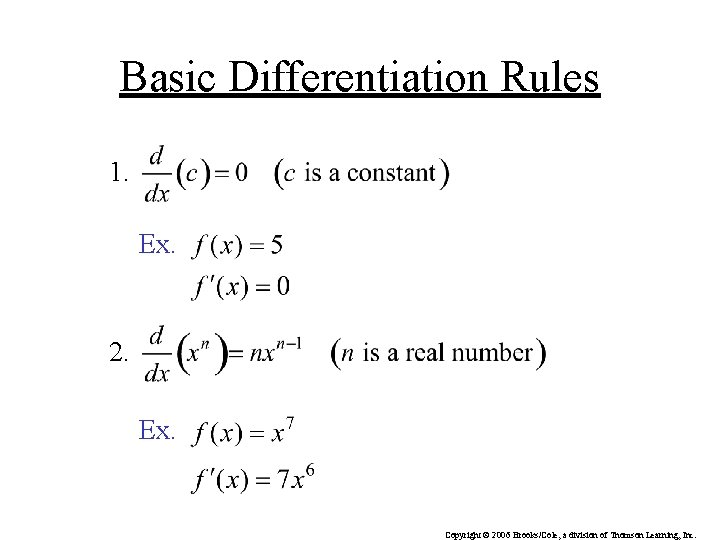Basic Differentiation Rules 1. Ex. 2. Ex. Copyright © 2006 Brooks/Cole, a division of Thomson Learning, Inc.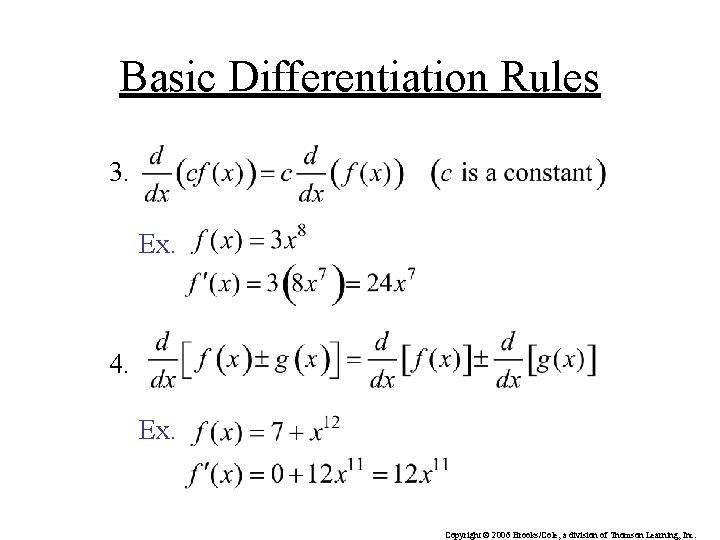Basic Differentiation Rules 3. Ex. 4. Ex. Copyright © 2006 Brooks/Cole, a division of Thomson Learning, Inc.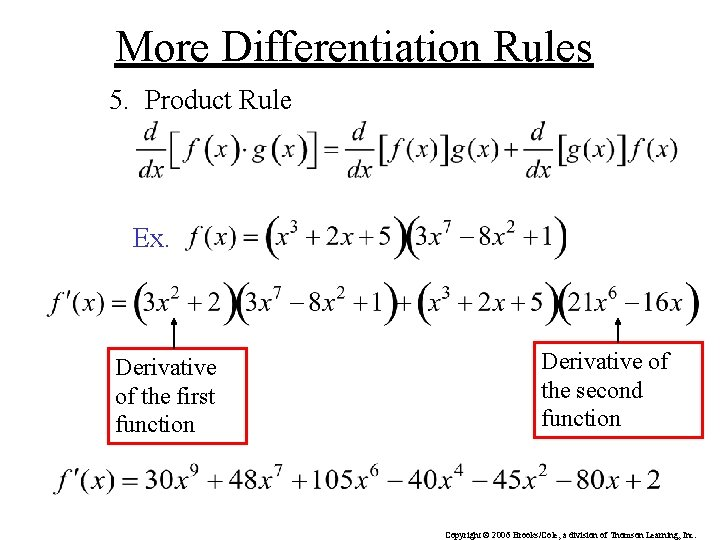More Differentiation Rules 5. Product Rule Ex. Derivative of the first function Derivative of the second function Copyright © 2006 Brooks/Cole, a division of Thomson Learning, Inc.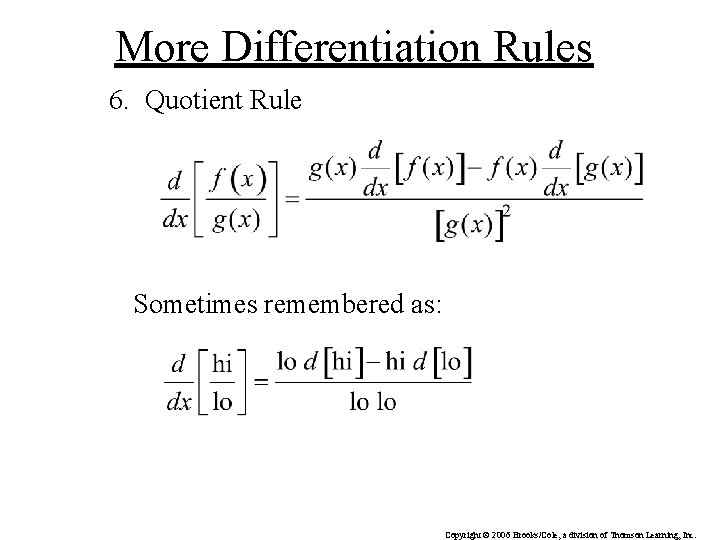More Differentiation Rules 6. Quotient Rule Sometimes remembered as: Copyright © 2006 Brooks/Cole, a division of Thomson Learning, Inc.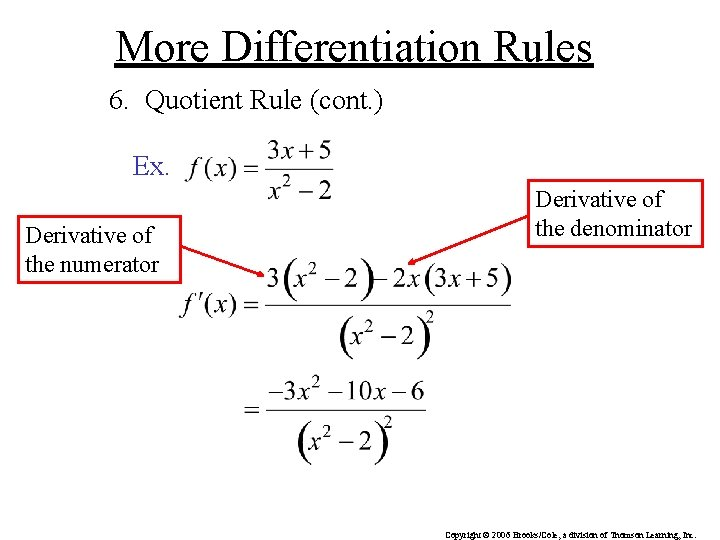More Differentiation Rules 6. Quotient Rule (cont. ) Ex. Derivative of the numerator Derivative of the denominator Copyright © 2006 Brooks/Cole, a division of Thomson Learning, Inc.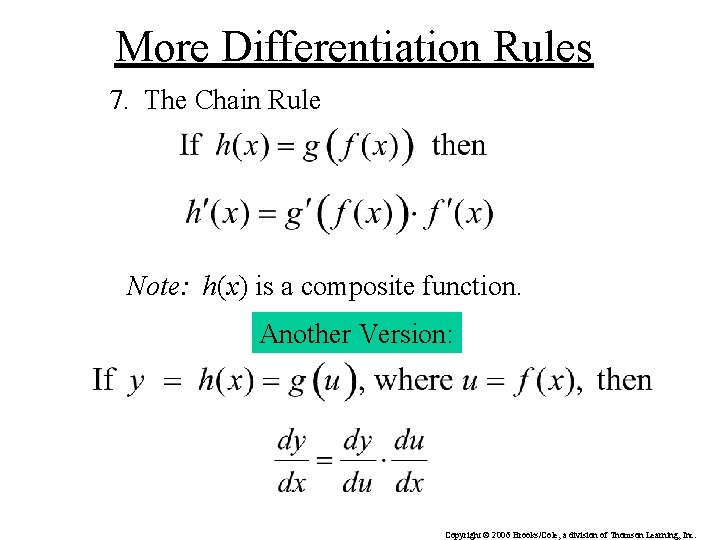More Differentiation Rules 7. The Chain Rule Note: h(x) is a composite function. Another Version: Copyright © 2006 Brooks/Cole, a division of Thomson Learning, Inc.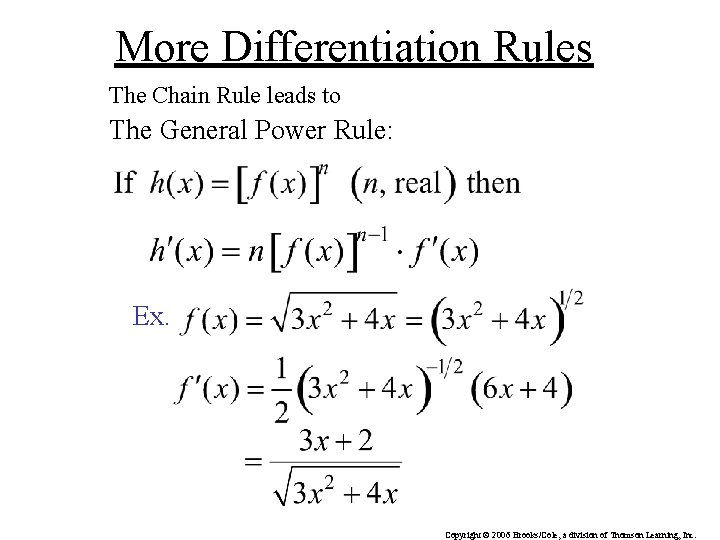More Differentiation Rules The Chain Rule leads to The General Power Rule: Ex. Copyright © 2006 Brooks/Cole, a division of Thomson Learning, Inc.Chain Rule Example Ex. Copyright © 2006 Brooks/Cole, a division of Thomson Learning, Inc.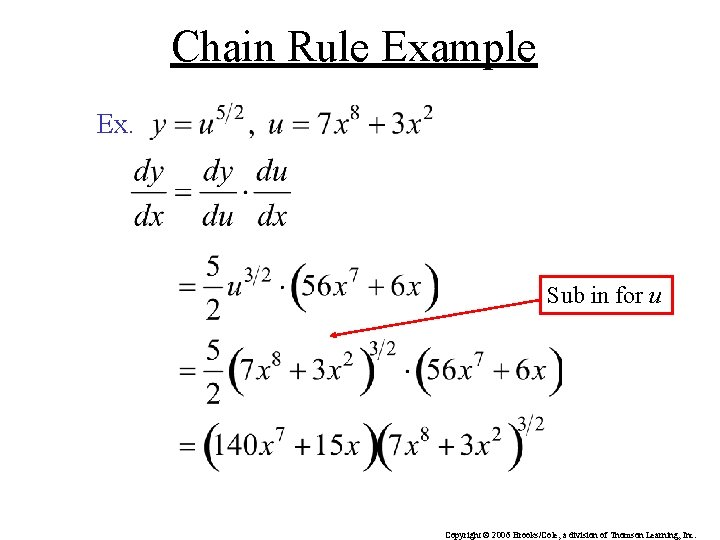Chain Rule Example Ex. Sub in for u Copyright © 2006 Brooks/Cole, a division of Thomson Learning, Inc.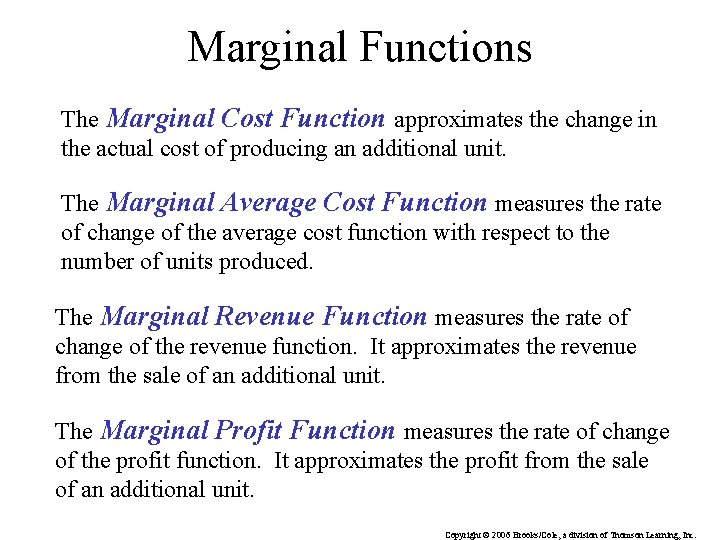Marginal Functions The Marginal Cost Function approximates the change in the actual cost of producing an additional unit. The Marginal Average Cost Function measures the rate of change of the average cost function with respect to the number of units produced. The Marginal Revenue Function measures the rate of change of the revenue function. It approximates the revenue from the sale of an additional unit. The Marginal Profit Function measures the rate of change of the profit function. It approximates the profit from the sale of an additional unit. Copyright © 2006 Brooks/Cole, a division of Thomson Learning, Inc.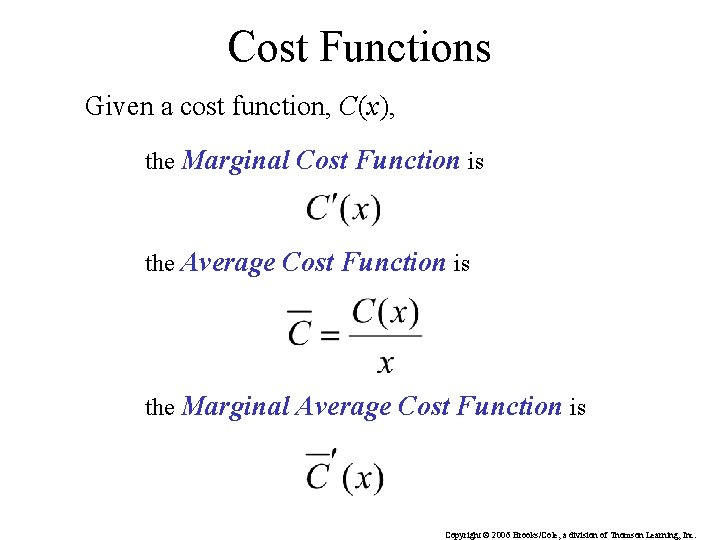Cost Functions Given a cost function, C(x), the Marginal Cost Function is the Average Cost Function is the Marginal Average Cost Function is Copyright © 2006 Brooks/Cole, a division of Thomson Learning, Inc.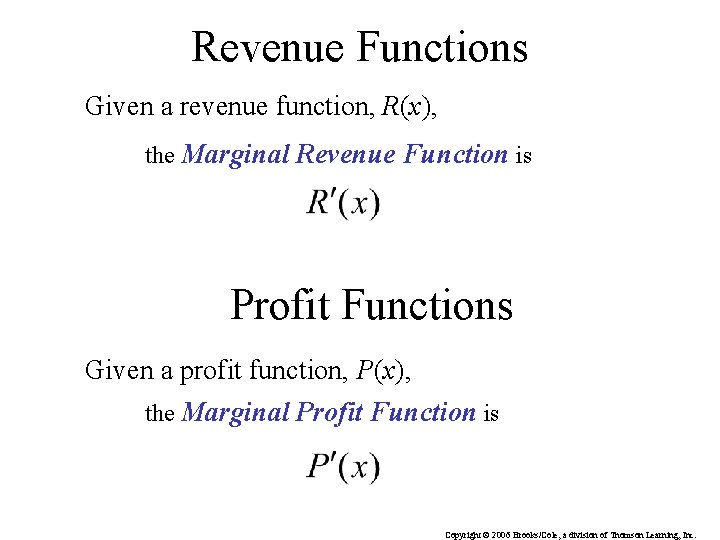Revenue Functions Given a revenue function, R(x), the Marginal Revenue Function is Profit Functions Given a profit function, P(x), the Marginal Profit Function is Copyright © 2006 Brooks/Cole, a division of Thomson Learning, Inc.Elasticity of Demand If f is a differentiable demand function defined by Then the elasticity of demand at price p is given by Demand is: Elastic if E(p) > 1 Unitary if E(p) = 1 Inelastic if E(p) < 1 Copyright © 2006 Brooks/Cole, a division of Thomson Learning, Inc.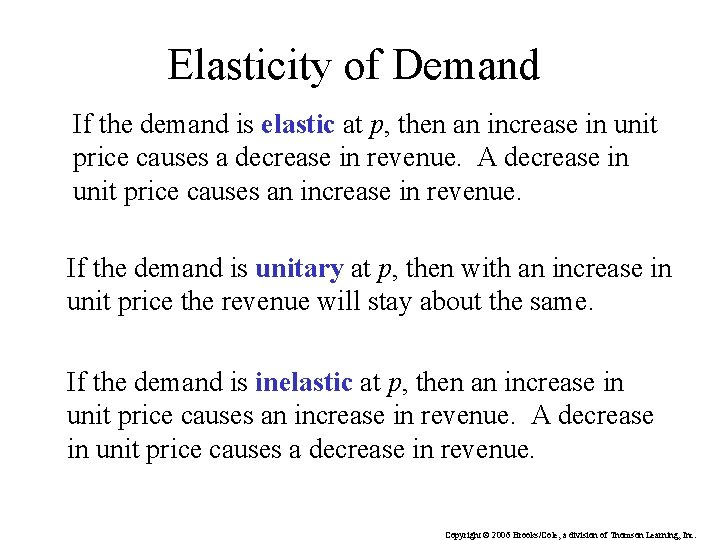Elasticity of Demand If the demand is elastic at p, then an increase in unit price causes a decrease in revenue. A decrease in unit price causes an increase in revenue. If the demand is unitary at p, then with an increase in unit price the revenue will stay about the same. If the demand is inelastic at p, then an increase in unit price causes an increase in revenue. A decrease in unit price causes a decrease in revenue. Copyright © 2006 Brooks/Cole, a division of Thomson Learning, Inc.Example Consider the demand equation which describes the relationship between the unit price p in dollars and the quantity demanded x of the Acrosonic model F loudspeaker systems. Find the elasticity of demand Copyright © 2006 Brooks/Cole, a division of Thomson Learning, Inc.Example The monthly demand for T-shirts is given by where p denotes the wholesale unit price in dollars and x denotes the quantity demanded. The monthly cost function for these T-shirts is 1. Find the revenue and profit functions. 2. Find the marginal cost, marginal revenue, and marginal profit functions. 3. Find the marginal average cost function. …… Copyright © 2006 Brooks/Cole, a division of Thomson Learning, Inc.Solution 1. Find the revenue and profit functions. Revenue = xp Profit = revenue – cost 2. Find the marginal cost, marginal revenue, and marginal profit functions. Marginal Cost = Copyright © 2006 Brooks/Cole, a division of Thomson Learning, Inc.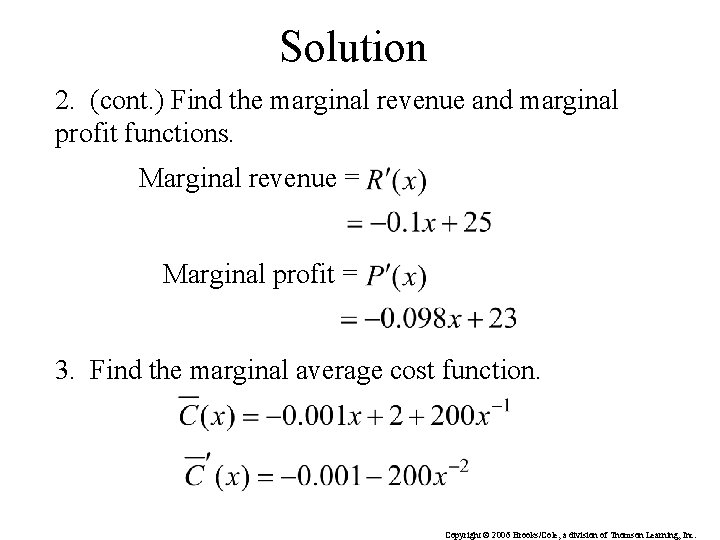Solution 2. (cont. ) Find the marginal revenue and marginal profit functions. Marginal revenue = Marginal profit = 3. Find the marginal average cost function. Copyright © 2006 Brooks/Cole, a division of Thomson Learning, Inc.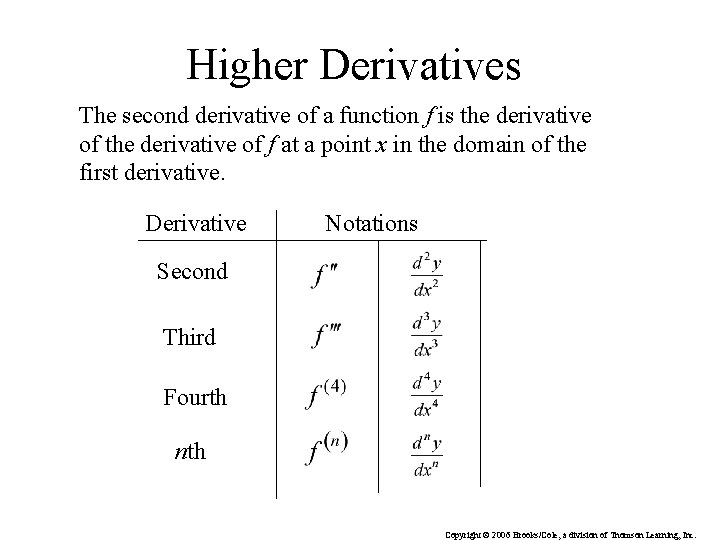Higher Derivatives The second derivative of a function f is the derivative of f at a point x in the domain of the first derivative. Derivative Notations Second Third Fourth nth Copyright © 2006 Brooks/Cole, a division of Thomson Learning, Inc.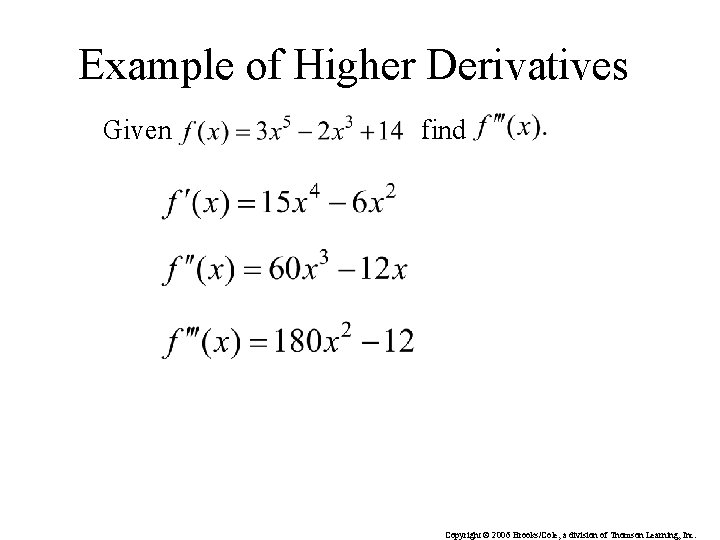Example of Higher Derivatives Given find Copyright © 2006 Brooks/Cole, a division of Thomson Learning, Inc.Example of Higher Derivatives Given find Copyright © 2006 Brooks/Cole, a division of Thomson Learning, Inc.Implicit Differentiation y is expressed explicitly as a function of x. y is expressed implicitly as a function of x. To differentiate the implicit equation, we write f (x) in place of y to get: Copyright © 2006 Brooks/Cole, a division of Thomson Learning, Inc.Implicit Differentiation (cont. ) Now differentiate using the chain rule: which can be written in the form subbing in y Solve for y’ Copyright © 2006 Brooks/Cole, a division of Thomson Learning, Inc.Related Rates Look at how the rate of change of one quantity is related to the rate of change of another quantity. Ex. Two cars leave an intersection at the same time. One car travels north at 35 mi. /hr. , the other travels west at 60 mi. /hr. How fast is the distance between them changing after 2 hours? Note: The rate of change of the distance between them is related to the rate at which the cars are traveling. Copyright © 2006 Brooks/Cole, a division of Thomson Learning, Inc.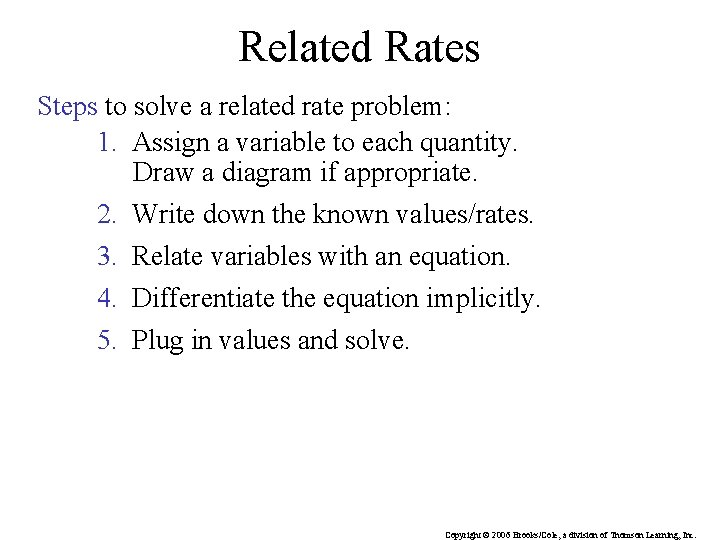Related Rates Steps to solve a related rate problem: 1. Assign a variable to each quantity. Draw a diagram if appropriate. 2. Write down the known values/rates. 3. Relate variables with an equation. 4. Differentiate the equation implicitly. 5. Plug in values and solve. Copyright © 2006 Brooks/Cole, a division of Thomson Learning, Inc.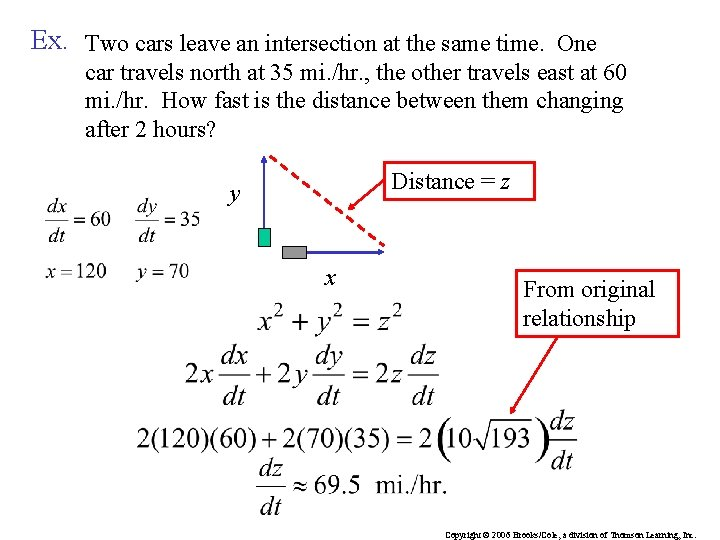Ex. Two cars leave an intersection at the same time. One car travels north at 35 mi. /hr. , the other travels east at 60 mi. /hr. How fast is the distance between them changing after 2 hours? Distance = z y x From original relationship Copyright © 2006 Brooks/Cole, a division of Thomson Learning, Inc.Increments An increment in x represents a change from x 1 to x 2 and is defined by: Read “delta x” An increment in y represents a change in y and is defined by: Copyright © 2006 Brooks/Cole, a division of Thomson Learning, Inc.Differentials Let y = f (x) be a differentiable function, then the differential of x, denoted dx, is such that The differential of y, denoted dy, is Note: Copyright © 2006 Brooks/Cole, a division of Thomson Learning, Inc.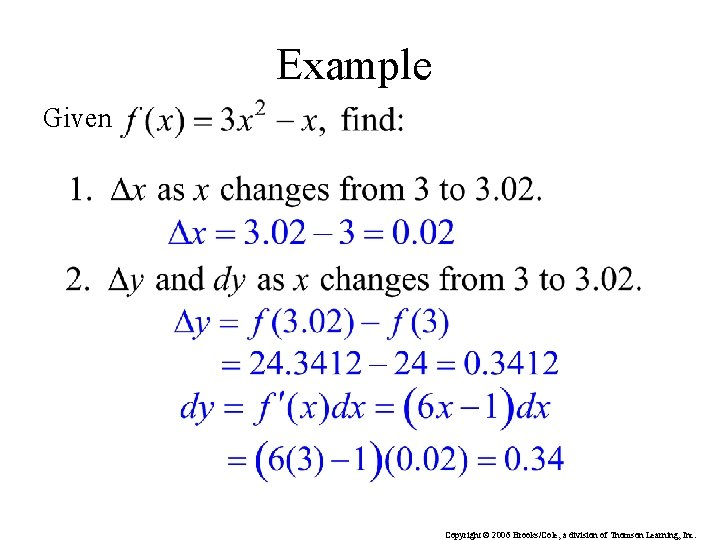Example Given Copyright © 2006 Brooks/Cole, a division of Thomson Learning, Inc.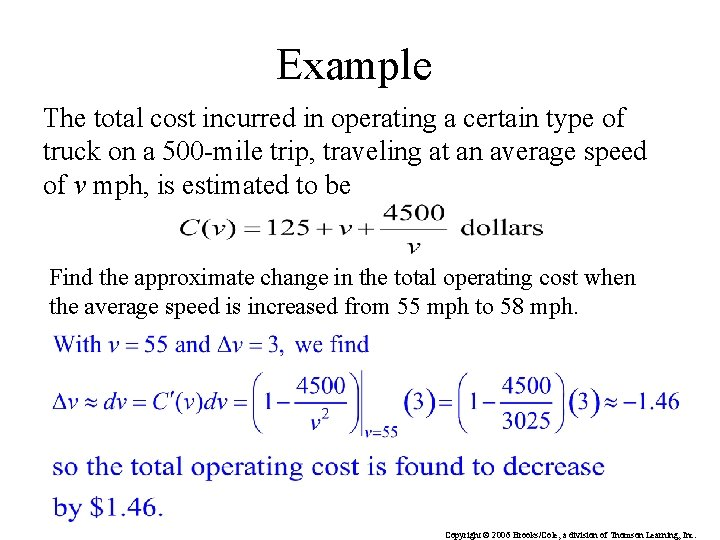Example The total cost incurred in operating a certain type of truck on a 500 -mile trip, traveling at an average speed of v mph, is estimated to be Find the approximate change in the total operating cost when the average speed is increased from 55 mph to 58 mph. Copyright © 2006 Brooks/Cole, a division of Thomson Learning, Inc.